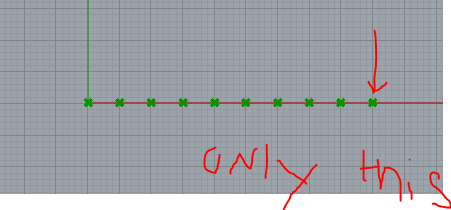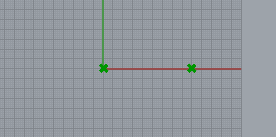# Moving a point by a single step by using series function

Hi,

I am trying to move a point by a single value which i am able to control. using the series function, i can move the point by an increment of 5 in the x direction. However, it moves the point depending on the count of the series. For example, when i want the point to x=45, i put my count to 10 (9*5=45). However, instead of moving the point only to that location, it moves it in all the previous steps as well. How do i make it so it only moves to x=45. I need to make it so the point only moves to the final point. So if the count is at 5, the point should only move to x=25.

Any ideas?

[moving point.gh|attachment]moving point.gh (5.0 KB)

use mass addition from the math functions to add all your values in the list together. What you are doing is multiplication, I doubt Series component is the best way to do it.

As you can see in the panel you conected after the series component, the output is a list as long as defined in the count input.
I guess what you want to do is use the multiplication component which naturally gives you only a*b as a result.

Yes but with the series component i am able to choose from the count, meaning i can choose the position of the point by moving the count. (in this example i can choose from 5, 10, 20…all the way up to 45 depending on what i need.). if i use multiplication i only get one value which i cannot change right?

I found this following method to work: By using the list item, i am able to choose the last value of the list which will give me position i want.I wonder if i could also do this by simply multiplying?

Hi -

If you use multiplication, you get one value. I think that’s what you want, no?

No, you can change both numbers that you use in the multiplication. And, if I understand it correctly, you only want to change one of these.-wim

1 Like

Ah i understand yh that would work as well! It would save memory as well which is better. Thanks a lot